Tuesday, March 28, 2023

# Discover How to Solve Math Problems With This Microsoft Math Solver App for Android

-

It is no secret to anyone that mathematics can become a real headache. However, there are many tools that can make your life easier. For this reason, we will explain how to solve mathematical problems with a Microsoft app for Android.

Microsoft Math Solver is able to help you with arithmetic, algebra, trigonometry, calculus, statistics and other issues using an advanced mathematical solver with Artificial Intelligence. Just write it on your mobile screen or take a picture of that problem you want to solve!

## So you can solve math problems with Math Solver

Microsoft Math Solver instantly recognizes the problem and helps you solve it with a step-by-step explanation, interactive graphics, similar web problems, and online video conferences. Mathematical problems are solved as follows:

• When you open it, you will see the Scan, Draw and Write options at the top. For this example, we will solve a mathematical problem with the function of Drawing.
• After clicking Draw, you will see a grid sheet on which you can write a mathematical equation as you naturally do on paper.
• Then press the arrow below for the application to do its magic and show you the result.

It is done! It’s that easy to solve math problems with Microsoft Math Solver. You can also do it by scanning a photo or importing an image with equations that you have saved in your gallery.•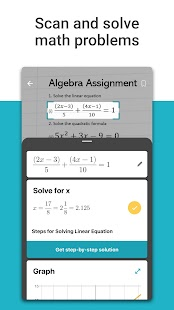•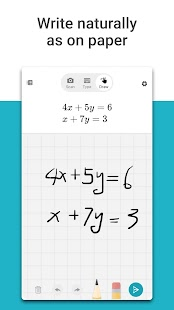•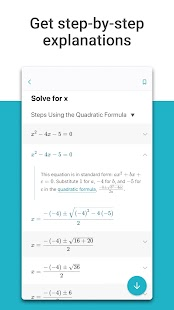•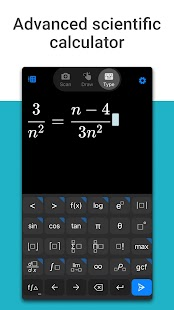•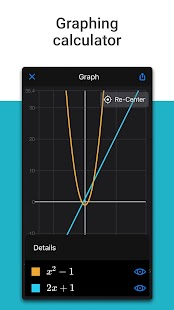•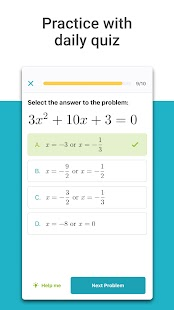••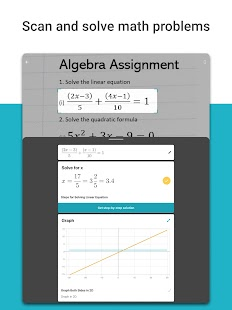•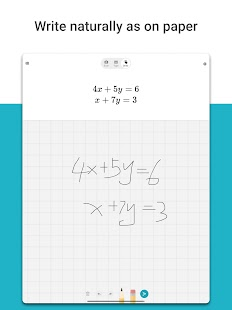•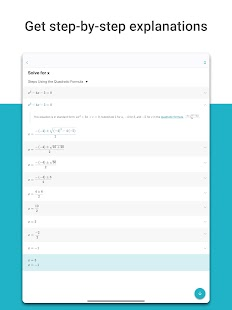•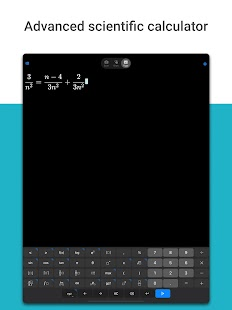•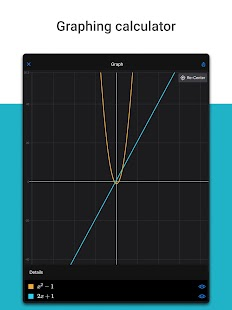•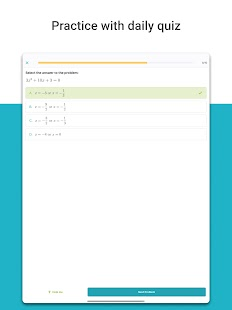•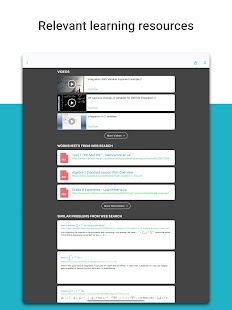•••••••## Issues supported by Microsoft Math Solver:

• Elemental: arithmetic, real, complex numbers, LCM, GCF, factors, Roman numerals.
• Pre-algebra: radicals and exponents, fractions, matrices, determinants.
• Algebra: quadratic equations, system of equations, inequalities, rational expressions, linear, quadratic and exponential graphs.
• Verbal problems about mathematical concepts, number theory, probability, volume, surface area.
• Basic calculation: sums, limits, derivatives, integrals.
• Statistics: mean, median, mode, standard deviation, permutations, combinations.

You realized? Microsoft Math Solver has everything you need to solve mathematical problems from your Android. Ideal for students, professionals or for anyone who needs a little help in their mathematical operations.Inclusive Post Journal is a Smart Journal, which provides internet viewers access to world news and real-life stories. Whether it is entertainment, Technology, Allrecipes, business, Insurance, Lifestyle, Travel or such.

This site uses Akismet to reduce spam. Learn how your comment data is processed.